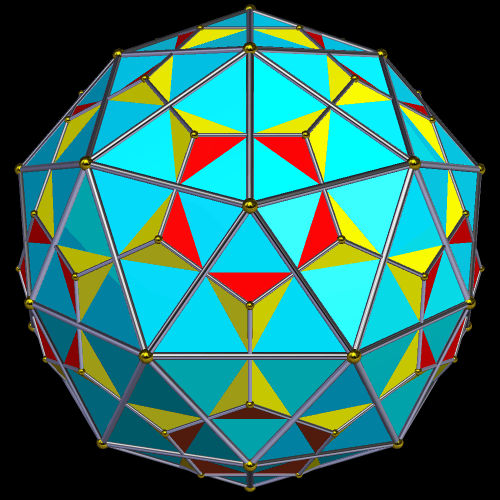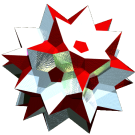Acronym pakid Name pentakis dodecahedron,apiculated dodecahedron©© (compound with its dual) Vertex figure [t6], [T5] Dihedral angles at long edge:   arccos[-(80+9 sqrt(5))/109] = 156.718554° at short edge:   arccos[-(80+9 sqrt(5))/109] = 156.718554° Dual ti ExternallinksThe triangles {(t,t,T)} have vertex angles t = arccos[(9-sqrt(5))/12] = 55.690640° resp. T = arccos[(9 sqrt(5)-7)/36] = 68.618721°.

Edge sizes used here are tT = x = 1 (short) resp. tt = a = (9-sqrt(5))/6 = 1.127322 (long).

Incidence matrix according to Dynkin symbol

m3m5o =
fo3oo5oa&#zx   → height = 0
a = (9-sqrt(5))/6 = 1.127322

o.3o.5o.    | 12  * |  5  0 |  5  [T5]
.o3.o5.o    |  * 20 |  3  3 |  6  [t6]
------------+-------+-------+---
oo3oo5oo&#x |  1  1 | 60  * |  2  x
.. .. .a    |  0  2 |  * 30 |  2  b
------------+-------+-------+---
.. .. oa&#x |  1  2 |  2  1 | 60  {(t,t,T)}